# Associated Legendre function

In mathematics and physics, an associated Legendre function Pm is related to a Legendre polynomial P by the following equation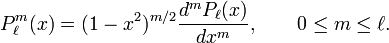$P^{m}_\ell(x) = (1-x^2)^{m/2} \frac{d^m P_\ell(x)}{dx^m}, \qquad 0 \le m \le \ell.$

Although extensions are possible, in this article and m are restricted to integer numbers. For even m the associated Legendre function is a polynomial, for odd m the function contains the factor (1−x ² )½ and hence is not a polynomial.

The associated Legendre functions are important in quantum mechanics and potential theory.

According to Ferrers the polynomials were named "Associated Legendre functions" by the British mathematician Isaac Todhunter in 1875, where "associated function" is Todhunter's translation of the German term zugeordnete Function, coined in 1861 by Heine, and "Legendre" is in honor of the French mathematician Adrien-Marie Legendre (1752–1833), who was the first to introduce and study the functions.

## Differential equation

Define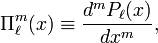$\Pi^{m}_\ell(x) \equiv \frac{d^m P_\ell(x)}{dx^m},$

where P(x) is a Legendre polynomial. Differentiating the Legendre differential equation: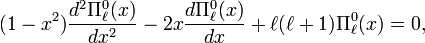$(1-x^2) \frac{d^2 \Pi^{0}_\ell(x)}{dx^2} - 2 x \frac{d\Pi^{0}_\ell(x)}{dx} + \ell(\ell+1) \Pi^{0}_\ell(x) = 0,$

m times gives an equation for Πml$(1-x^2) \frac{d^2 \Pi^{m}_\ell(x)}{dx^2} - 2(m+1) x \frac{d\Pi^{m}_\ell(x)}{dx} + \left[\ell(\ell+1) -m(m+1) \right] \Pi^{m}_\ell(x) = 0 .$

After substitution of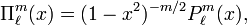$\Pi^{m}_\ell(x) = (1-x^2)^{-m/2} P^{m}_\ell(x),$

and after multiplying through with (1 − x2)m / 2, we find the associated Legendre differential equation: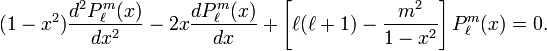$(1-x^2) \frac{d^2 P^{m}_\ell(x)}{dx^2} -2x\frac{d P^{m}_\ell(x)}{dx} + \left[ \ell(\ell+1) - \frac{m^2}{1-x^2}\right] P^{m}_\ell(x)= 0 .$

One often finds the equation written in the following equivalent way$\left( (1-x^{2})\; y\,' \right)' +\left( \ell(\ell+1) -\frac{m^{2} }{1-x^{2} } \right) y=0,$

where the primes indicate differentiation with respect to x.

In physical applications it is usually the case that x = cosθ, then the associated Legendre differential equation takes the form$\frac{1}{\sin \theta}\frac{d}{d\theta} \sin\theta \frac{d}{d\theta}P^{m}_\ell +\left[ \ell(\ell+1) - \frac{m^2}{\sin^2\theta}\right] P^{m}_\ell = 0.$

## Extension to negative m

By the Rodrigues formula, one obtains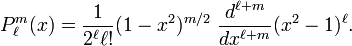$P_\ell^{m}(x) = \frac{1}{2^\ell \ell!} (1-x^2)^{m/2}\ \frac{d^{\ell+m}}{dx^{\ell+m}}(x^2-1)^\ell.$

This equation allows extension of the range of m to: −mm.

Since the associated Legendre equation is invariant under the substitution m → −m, the equations for P ±m, resulting from this expression, are proportional.

To obtain the proportionality constant we consider$(1-x^2)^{-m/2} \frac{d^{\ell-m}}{dx^{\ell-m}} (x^2-1)^{\ell} = c_{lm} (1-x^2)^{m/2} \frac{d^{\ell+m}}{dx^{\ell+m}}(x^2-1)^{\ell},\qquad 0 \le m \le \ell,$

and we bring the factor (1−x²)m/2 to the other side. Equate the coefficient of the highest power of x on the left and right hand side of$\frac{d^{\ell-m}}{dx^{\ell-m}} (x^2-1)^{\ell} = c_{lm} (1-x^2)^m \frac{d^{\ell+m}}{dx^{\ell+m}}(x^2-1)^{\ell},\qquad 0 \le m \le \ell,$

and it follows that the proportionality constant is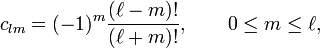$c_{lm} = (-1)^m \frac{(\ell-m)!}{(\ell+m)!} ,\qquad 0 \le m \le \ell,$

so that the associated Legendre functions of same |m| are related to each other by$P^{-|m|}_\ell(x) = (-1)^m \frac{(\ell-|m|)!}{(\ell+|m|)!} P^{|m|}_\ell(x).$

Note that the phase factor (−1)m arising in this expression is not due to some arbitrary phase convention, but arises from expansion of (1−x²)m.

## Orthogonality relations

Important integral relations are:$\int\limits_{-1}^{1}P_{l}^{m} \left( x\right) P_{k}^{m} \left( x\right) dx =\frac{2}{2l+1} \frac{\left( l+m\right) !}{\left( l-m\right) !} \delta _{lk},$

and: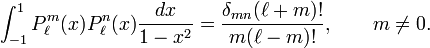$\int_{-1}^{1} P^{m}_{\ell}(x) P^{n}_{\ell}(x) \frac{d x}{1-x^2} = \frac{\delta_{mn}(\ell+m)!}{m(\ell-m)!}, \qquad m \ne 0 .$

The latter integral for n = m = 0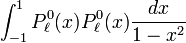$\int_{-1}^{1} P^{0}_{\ell}(x) P^{0}_{\ell}(x) \frac{d x}{1-x^2}$

is undetermined (infinite). (see Associated_Legendre_function/Proofs for detailed proofs of these relations.)

## Recurrence relations

The functions satisfy the following difference equations, which are taken from Edmonds.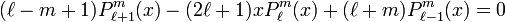$(\ell-m+1)P_{\ell+1}^{m}(x) - (2\ell+1)xP_{\ell}^{m}(x) + (\ell+m)P_{\ell-1}^{m}(x)=0$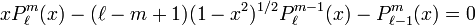$xP_{\ell}^{m}(x) -(\ell-m+1)(1-x^2)^{1/2} P_{\ell}^{m-1}(x) - P_{\ell-1}^{m}(x)=0$$P_{\ell+1}^{m}(x) - x P_{\ell}^{m}(x)-(\ell+m)(1-x^2)^{1/2}P_{\ell}^{m-1}(x)=0$$(\ell-m+1)P_{\ell+1}^{m}(x)+(1-x^2)^{1/2}P_{\ell}^{m+1}(x)- (\ell+m+1) xP_{\ell}^{m}(x)=0$$(1-x^2)^{1/2}P_{\ell}^{m+1}(x)-2mxP_{\ell}^{m}(x)+ (\ell+m)(\ell-m+1)(1-x^2)^{1/2}P_{\ell}^{m-1}(x)=0$$(1-x^2)\frac{dP_{\ell}^{m}}{dx}(x) =(\ell+1)xP_{\ell}^{m}(x) -(\ell-m+1)P_{\ell+1}^{m}(x)$$=(\ell+m)P_{\ell-1}^{m}(x)-\ell x P_{\ell}^{m}(x)$

## Reference

1. N. M. Ferrers, An Elementary Treatise on Spherical Harmonics, MacMillan, 1877 (London), p. 77. Online.
2. I. Todhunter, An Elementary Treatise on Laplace's, Lamé's, and Bessel's Functions, MacMillan, 1875 (London). In fact, Todhunter called the Legendre polynomials "Legendre coefficients".
3. E. Heine, Handbuch der Kugelfunctionen, G. Reimer, 1861 (Berlin).Google book online
4. The associated Legendre differential equation being of second order, the general solution is of the form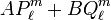$AP_\ell^m + BQ_\ell^m$ where$Q_\ell^m$ is a Legendre polynomial of the second kind, which has a singularity at x = 0. Hence solutions that are regular at x = 0 have B = 0 and are proportional to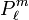$P_\ell^m$. The Rodrigues formula shows that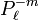$P_\ell^{-m}$ is a regular (at x=0) solution and the proportionality follows.
5. A. R. Edmonds, Angular Momentum in Quantum Mechanics, Princeton University Press, 2nd edition (1960)